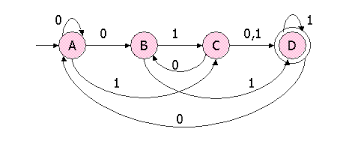# Unit 4 - Regular Languages And Finite Automaton Quiz

10 Questions | Attempts: 487SettingsATLS is the standard of care for trauma patients, and it is built around a consistent approach to patient evaluation. This protocol ensures that the most immediate life-threatening conditions are quickly identified and addressed in the order of their risk potential. Take the test and see how prepared you are for such an occurrence.

• 1.
The main difference between a DFSA and an NDFSA is
• A.

In DFSA, ε transition may be present

• B.

In NDFSA, ε transitions may be present

• C.

In DFSA, from any given state, there can't be any alphabet leading to two diferent states

• D.

In NDFSA, from any given state, there can't be any alphabet leading to two diferent states

• 2.
The major difference between a moore and mealy machine is that
• A.

Output of the former depends on the present state and present input

• B.

Output of the former depends only on the present state

• C.

Output of former depends only on the present input

• D.

All of these

• 3.
Any given transition graph has an equivalent
• A.

Regular expression

• B.

DFSM

• C.

NDFSM

• D.

All of these

• 4.
For which of the following application, regular expressions cannot be used ?
• A.

Designing computers

• B.

Designing compilers

• C.

Both (a) and (b)

• D.

Developing computers

• 5.
Which of the following regular expressions denotes a language comprising all possible strings over the alphabet {a, b } ?
• A.

A* b*

• B.

(a | b)*

• C.

(ab)+

• D.

(a | b*)

• 6.
Palindromes can't be recognized by any FSM because
• A.

FSM can't remember arbitrarily large of information

• B.

FSM can't deterministically fix the mid-point

• C.

Even if mid-point is known, FSM be can't be found whether, second half of the string matches the first half

• D.

All of these

• 7.
A language L is accepted by a finite automaton if and only if it is
• A.

Context - free

• B.

Context-sensitive

• C.

Recursive

• D.

Right-linear

• 8.
Can a DFA simulate NFA?
• A.

NO

• B.

YES

• C.

SOMETIMES

• D.

Depends on NFA

• 9.
Regular expression a / b denotes the set
• A.

{a}

• B.

{ ∈ , a, b }

• C.

{a, b}

• D.

{ ab }

• 10.
Regular expression (a | b ) (a | b) denotes the set
• A.

{ a, b, ab, aa }

• B.

{ a, b, ba, bb }

• C.

{ a, b }

• D.

{ aa, ab, ba, bb }

## Related TopicsBack to top